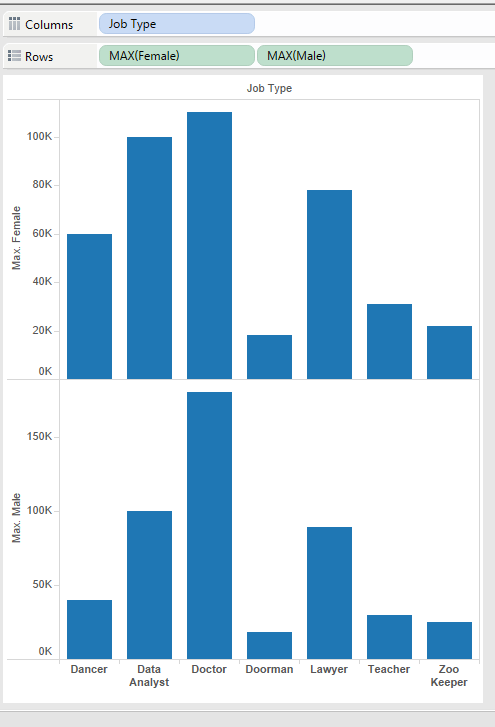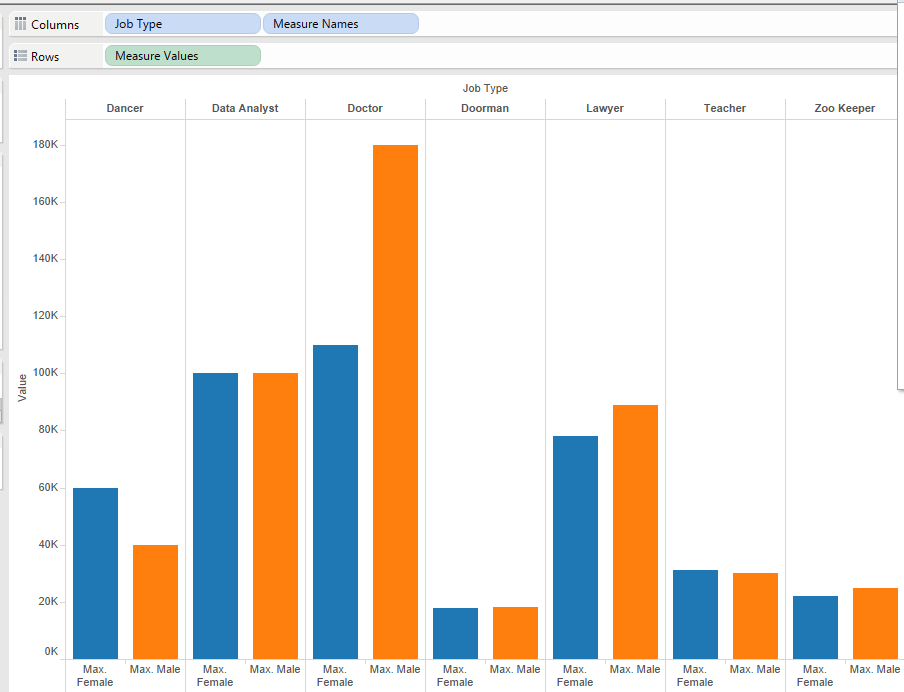# Tableau Dimensions and Measures 101

Tableau allows you to create amazing data visualizations using continuous and discrete variables. Otherwise known and columns and rows or dimensions and measures. We want to use these to define what you are measuring and how to measure it. For example, if I wanted to measure the website visits from different channels, your dimensions would be  what you are measuring. This would be the visits. These visits are a continuous variable from 1 to 1000 and the different channels are categorical variables known as dimensions.

To sum it up the category that you want to measure is a dimension and the measurement is the measures. However, please note that some variables can be both dimensions and measures.

Here is a quick quiz: Choose the where you would put these variables

Salary

Job Title

We know that salaries can have a wide continuous range. Imagine we are looking at salaries from \$18,000 per year to 118,000 dollars per year.  That means that we will start with \$18,000, 18,001 to 18,002  and so and so.  These variables would fall under measures.We want to breakup that by categories of job types. This will require you to place a dimension into the column of TableauWe can also divide the data using a separate measures.  Imagine if we wanted to see if the is a disparity in pay for job types between women and men. We can add two sets of continuous data( male salaries and females salaries). This can be categorized by either male or female.

Adding more than one measure to one individual dimension will divide the data. Using this option allows you to split the visualization into two separate charts by showing the relationship each measure has to the dimension.To improve the visualization, you can use a side by side comparison using color. This allows you to see the difference side by side.